Скачать презентацию Cryptography CS 555 Topic 23 Zero-Knowledge Proof and

• Количество слайдов: 40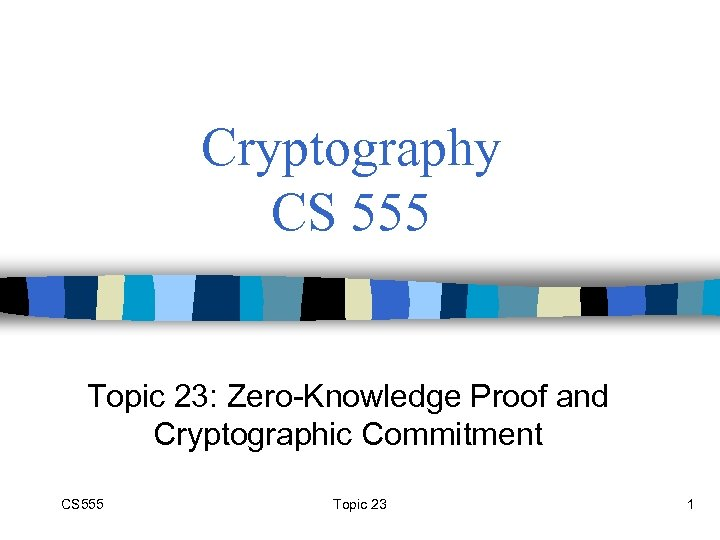Cryptography CS 555 Topic 23: Zero-Knowledge Proof and Cryptographic Commitment CS 555 Topic 23 1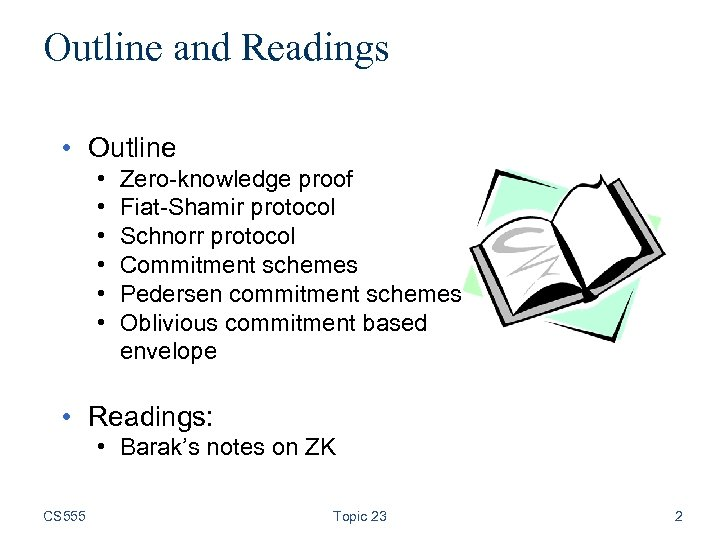Outline and Readings • Outline • • • Zero-knowledge proof Fiat-Shamir protocol Schnorr protocol Commitment schemes Pedersen commitment schemes Oblivious commitment based envelope • Readings: • Barak’s notes on ZK CS 555 Topic 23 2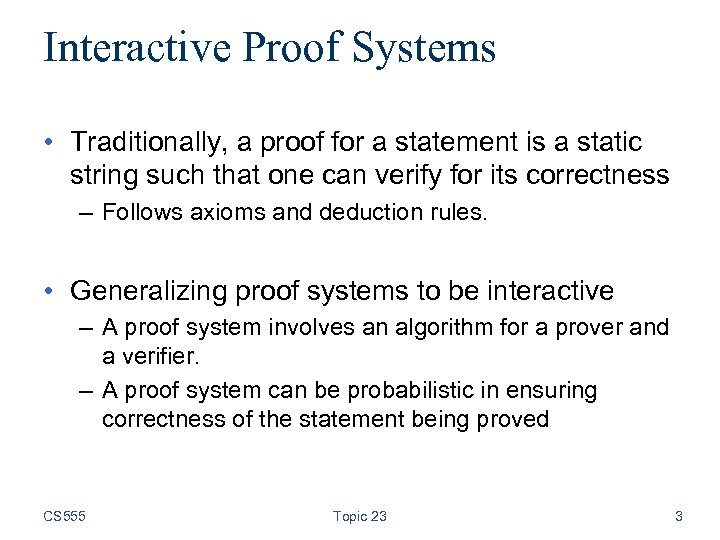Interactive Proof Systems • Traditionally, a proof for a statement is a static string such that one can verify for its correctness – Follows axioms and deduction rules. • Generalizing proof systems to be interactive – A proof system involves an algorithm for a prover and a verifier. – A proof system can be probabilistic in ensuring correctness of the statement being proved CS 555 Topic 23 3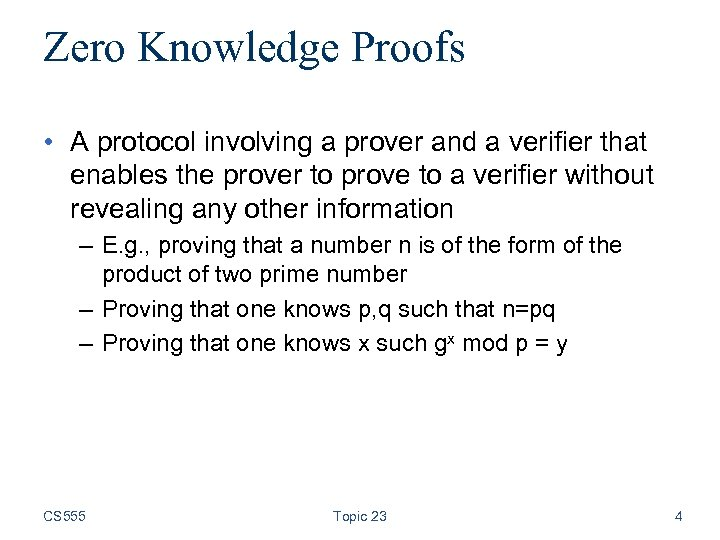Zero Knowledge Proofs • A protocol involving a prover and a verifier that enables the prover to prove to a verifier without revealing any other information – E. g. , proving that a number n is of the form of the product of two prime number – Proving that one knows p, q such that n=pq – Proving that one knows x such gx mod p = y CS 555 Topic 23 4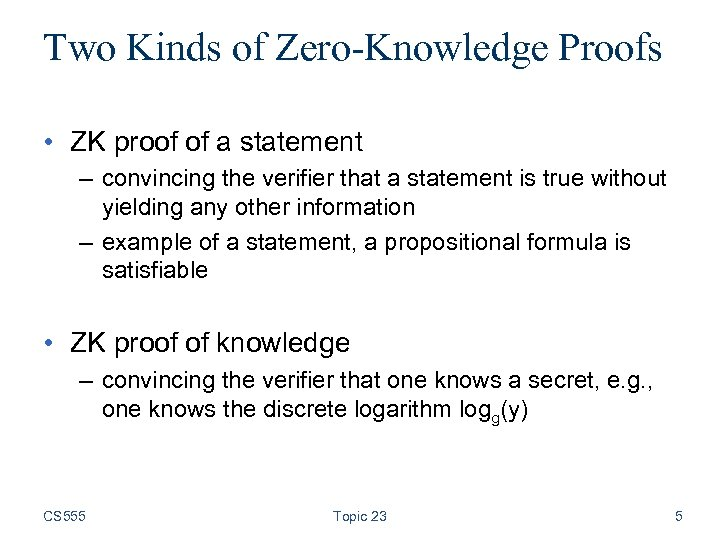Two Kinds of Zero-Knowledge Proofs • ZK proof of a statement – convincing the verifier that a statement is true without yielding any other information – example of a statement, a propositional formula is satisfiable • ZK proof of knowledge – convincing the verifier that one knows a secret, e. g. , one knows the discrete logarithm logg(y) CS 555 Topic 23 5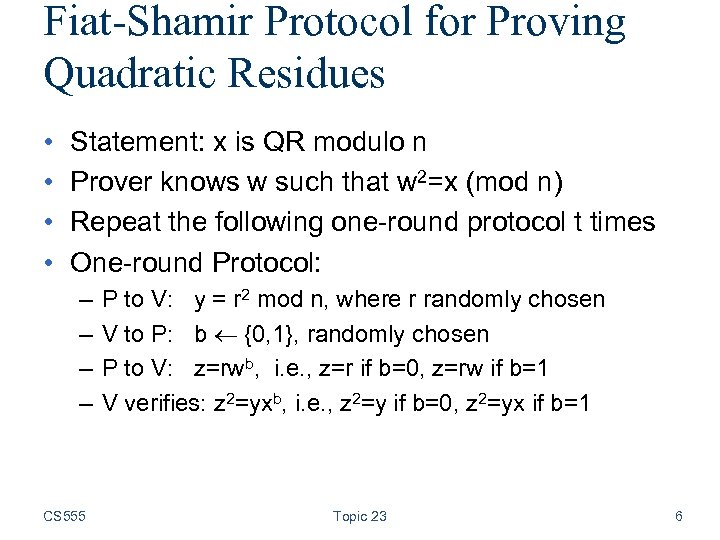Fiat-Shamir Protocol for Proving Quadratic Residues • • Statement: x is QR modulo n Prover knows w such that w 2=x (mod n) Repeat the following one-round protocol t times One-round Protocol: – – CS 555 P to V: y = r 2 mod n, where r randomly chosen V to P: b {0, 1}, randomly chosen P to V: z=rwb, i. e. , z=r if b=0, z=rw if b=1 V verifies: z 2=yxb, i. e. , z 2=y if b=0, z 2=yx if b=1 Topic 23 6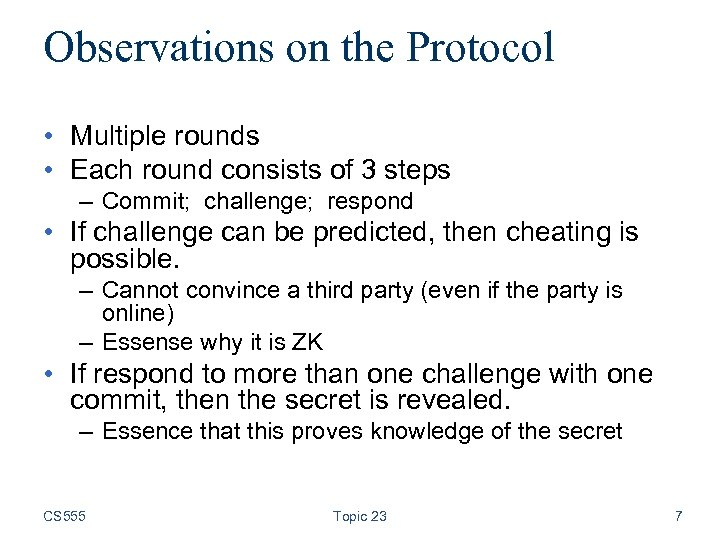Observations on the Protocol • Multiple rounds • Each round consists of 3 steps – Commit; challenge; respond • If challenge can be predicted, then cheating is possible. – Cannot convince a third party (even if the party is online) – Essense why it is ZK • If respond to more than one challenge with one commit, then the secret is revealed. – Essence that this proves knowledge of the secret CS 555 Topic 23 7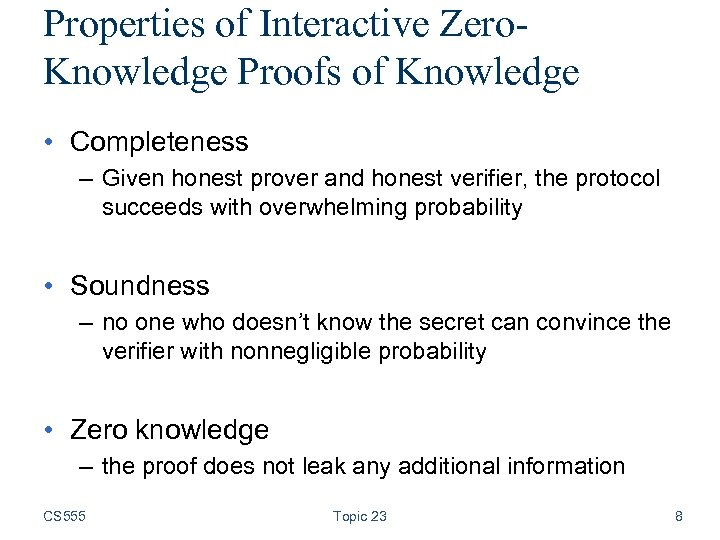Properties of Interactive Zero. Knowledge Proofs of Knowledge • Completeness – Given honest prover and honest verifier, the protocol succeeds with overwhelming probability • Soundness – no one who doesn’t know the secret can convince the verifier with nonnegligible probability • Zero knowledge – the proof does not leak any additional information CS 555 Topic 23 8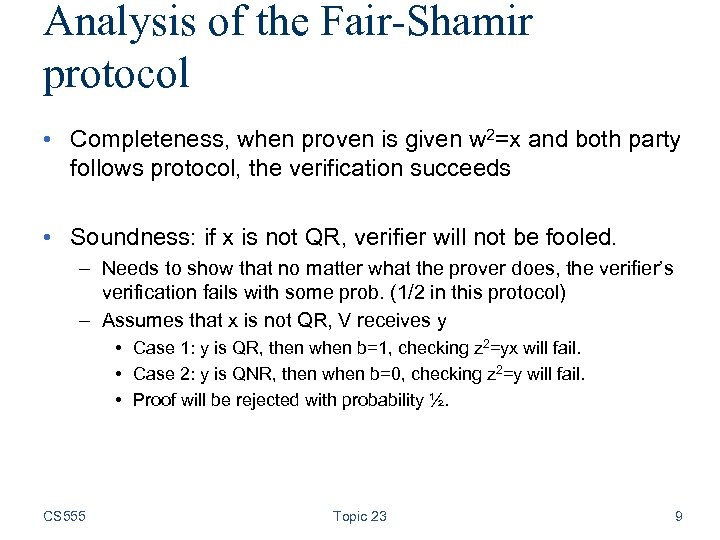Analysis of the Fair-Shamir protocol • Completeness, when proven is given w 2=x and both party follows protocol, the verification succeeds • Soundness: if x is not QR, verifier will not be fooled. – Needs to show that no matter what the prover does, the verifier’s verification fails with some prob. (1/2 in this protocol) – Assumes that x is not QR, V receives y • Case 1: y is QR, then when b=1, checking z 2=yx will fail. • Case 2: y is QNR, then when b=0, checking z 2=y will fail. • Proof will be rejected with probability ½. CS 555 Topic 23 9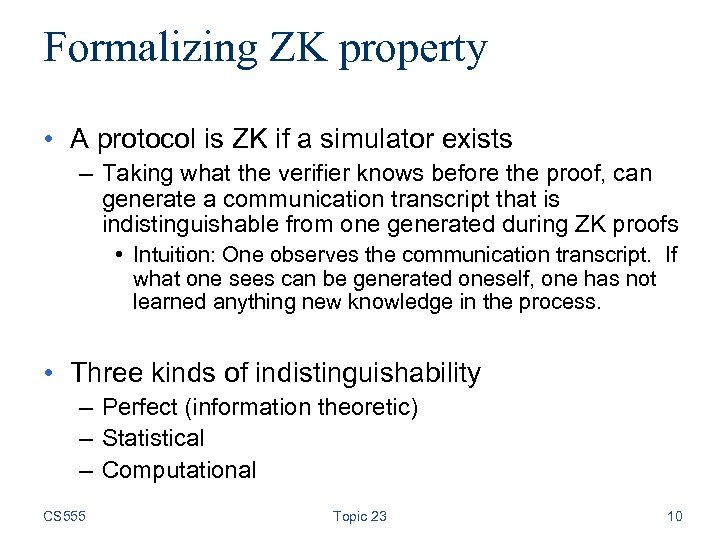Formalizing ZK property • A protocol is ZK if a simulator exists – Taking what the verifier knows before the proof, can generate a communication transcript that is indistinguishable from one generated during ZK proofs • Intuition: One observes the communication transcript. If what one sees can be generated oneself, one has not learned anything new knowledge in the process. • Three kinds of indistinguishability – Perfect (information theoretic) – Statistical – Computational CS 555 Topic 23 10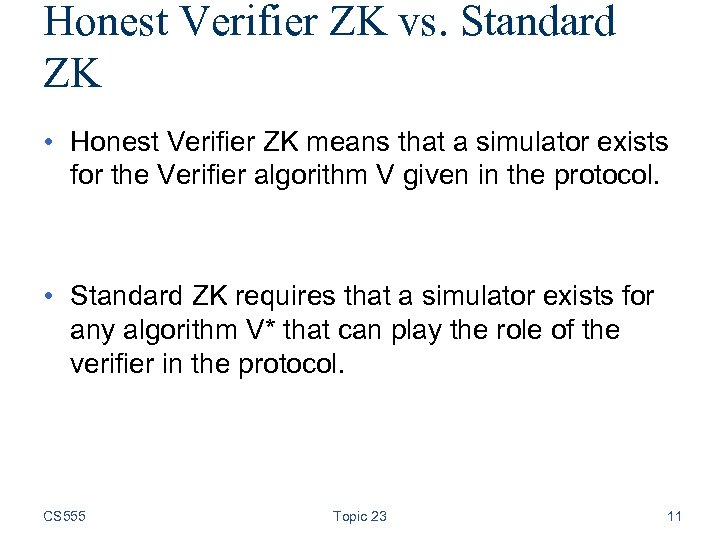Honest Verifier ZK vs. Standard ZK • Honest Verifier ZK means that a simulator exists for the Verifier algorithm V given in the protocol. • Standard ZK requires that a simulator exists for any algorithm V* that can play the role of the verifier in the protocol. CS 555 Topic 23 11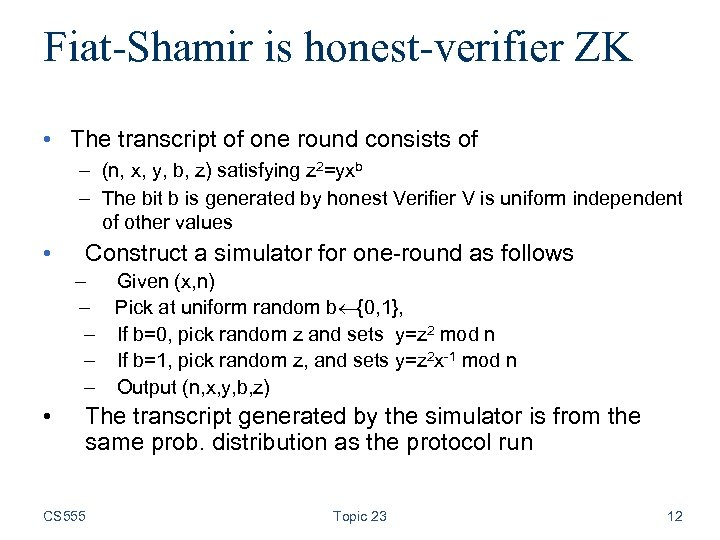Fiat-Shamir is honest-verifier ZK • The transcript of one round consists of – (n, x, y, b, z) satisfying z 2=yxb – The bit b is generated by honest Verifier V is uniform independent of other values • Construct a simulator for one-round as follows – Given (x, n) – Pick at uniform random b {0, 1}, – If b=0, pick random z and sets y=z 2 mod n – If b=1, pick random z, and sets y=z 2 x-1 mod n – Output (n, x, y, b, z) • The transcript generated by the simulator is from the same prob. distribution as the protocol run CS 555 Topic 23 12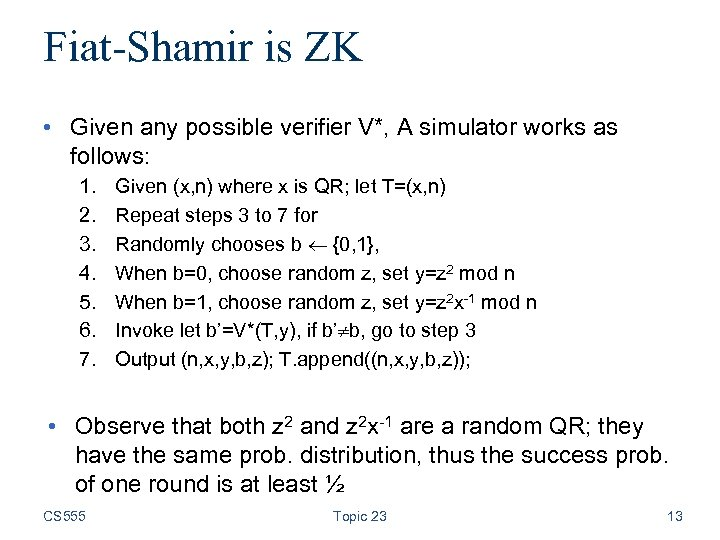Fiat-Shamir is ZK • Given any possible verifier V*, A simulator works as follows: 1. 2. 3. 4. 5. 6. 7. Given (x, n) where x is QR; let T=(x, n) Repeat steps 3 to 7 for Randomly chooses b {0, 1}, When b=0, choose random z, set y=z 2 mod n When b=1, choose random z, set y=z 2 x-1 mod n Invoke let b’=V*(T, y), if b’ b, go to step 3 Output (n, x, y, b, z); T. append((n, x, y, b, z)); • Observe that both z 2 and z 2 x-1 are a random QR; they have the same prob. distribution, thus the success prob. of one round is at least ½ CS 555 Topic 23 13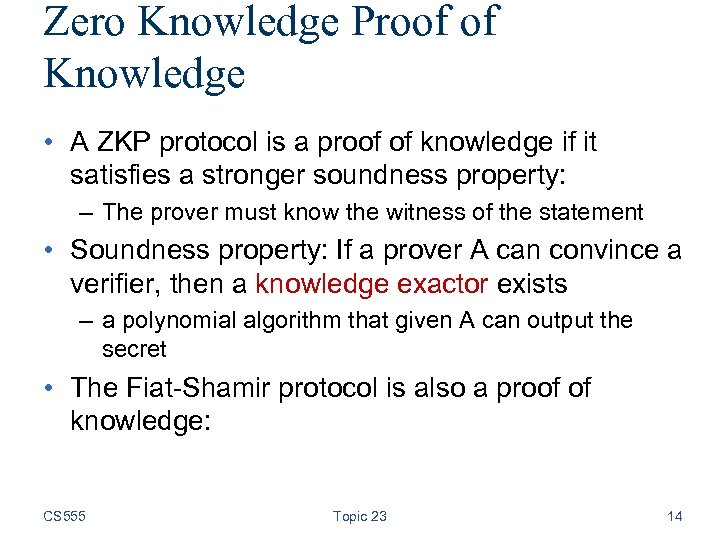Zero Knowledge Proof of Knowledge • A ZKP protocol is a proof of knowledge if it satisfies a stronger soundness property: – The prover must know the witness of the statement • Soundness property: If a prover A can convince a verifier, then a knowledge exactor exists – a polynomial algorithm that given A can output the secret • The Fiat-Shamir protocol is also a proof of knowledge: CS 555 Topic 23 14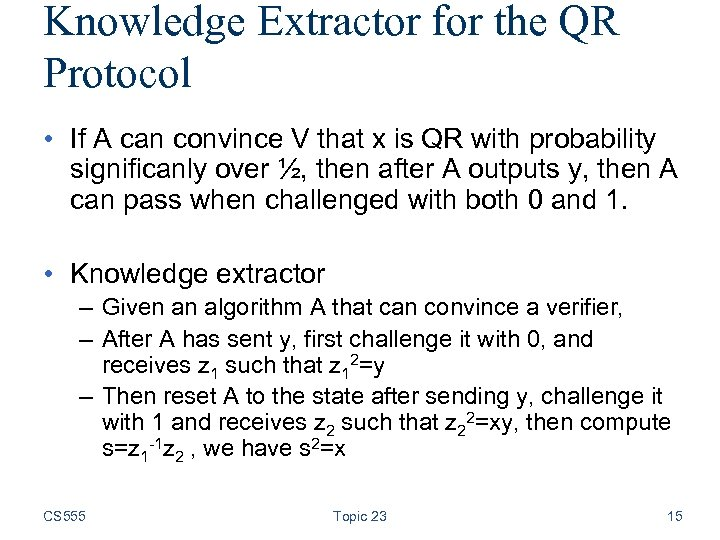Knowledge Extractor for the QR Protocol • If A can convince V that x is QR with probability significanly over ½, then after A outputs y, then A can pass when challenged with both 0 and 1. • Knowledge extractor – Given an algorithm A that can convince a verifier, – After A has sent y, first challenge it with 0, and receives z 1 such that z 12=y – Then reset A to the state after sending y, challenge it with 1 and receives z 2 such that z 22=xy, then compute s=z 1 -1 z 2 , we have s 2=x CS 555 Topic 23 15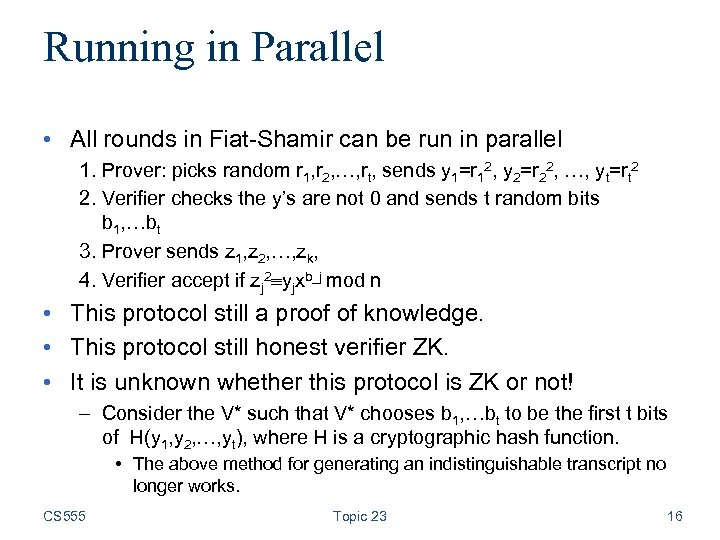Running in Parallel • All rounds in Fiat-Shamir can be run in parallel 1. Prover: picks random r 1, r 2, …, rt, sends y 1=r 12, y 2=r 22, …, yt=rt 2 2. Verifier checks the y’s are not 0 and sends t random bits b 1, …bt 3. Prover sends z 1, z 2, …, zk, 4. Verifier accept if zj 2 yjxb_j mod n • This protocol still a proof of knowledge. • This protocol still honest verifier ZK. • It is unknown whether this protocol is ZK or not! – Consider the V* such that V* chooses b 1, …bt to be the first t bits of H(y 1, y 2, …, yt), where H is a cryptographic hash function. • The above method for generating an indistinguishable transcript no longer works. CS 555 Topic 23 16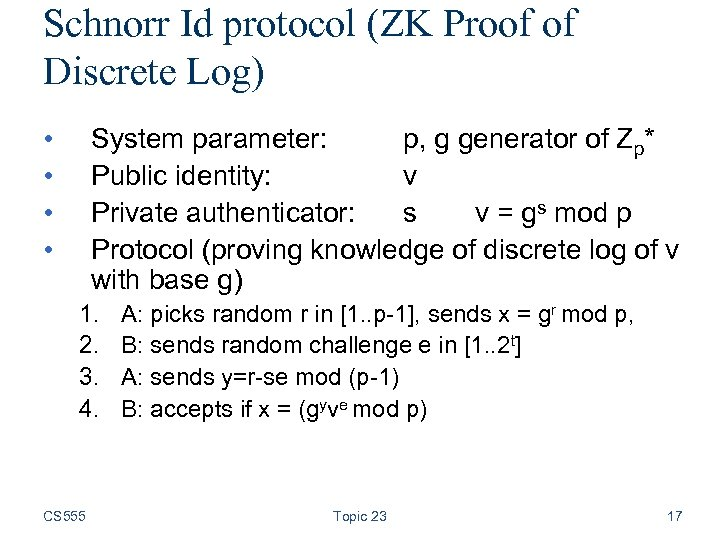Schnorr Id protocol (ZK Proof of Discrete Log) • • System parameter: p, g generator of Zp* Public identity: v Private authenticator: s v = gs mod p Protocol (proving knowledge of discrete log of v with base g) 1. 2. 3. 4. CS 555 A: picks random r in [1. . p-1], sends x = gr mod p, B: sends random challenge e in [1. . 2 t] A: sends y=r-se mod (p-1) B: accepts if x = (gyve mod p) Topic 23 17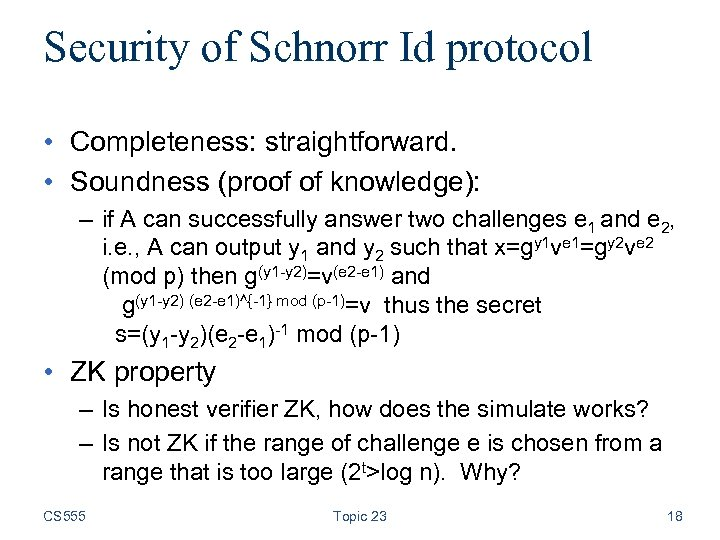Security of Schnorr Id protocol • Completeness: straightforward. • Soundness (proof of knowledge): – if A can successfully answer two challenges e 1 and e 2, i. e. , A can output y 1 and y 2 such that x=gy 1 ve 1=gy 2 ve 2 (mod p) then g(y 1 -y 2)=v(e 2 -e 1) and g(y 1 -y 2) (e 2 -e 1)^{-1} mod (p-1)=v thus the secret s=(y 1 -y 2)(e 2 -e 1)-1 mod (p-1) • ZK property – Is honest verifier ZK, how does the simulate works? – Is not ZK if the range of challenge e is chosen from a range that is too large (2 t>log n). Why? CS 555 Topic 23 18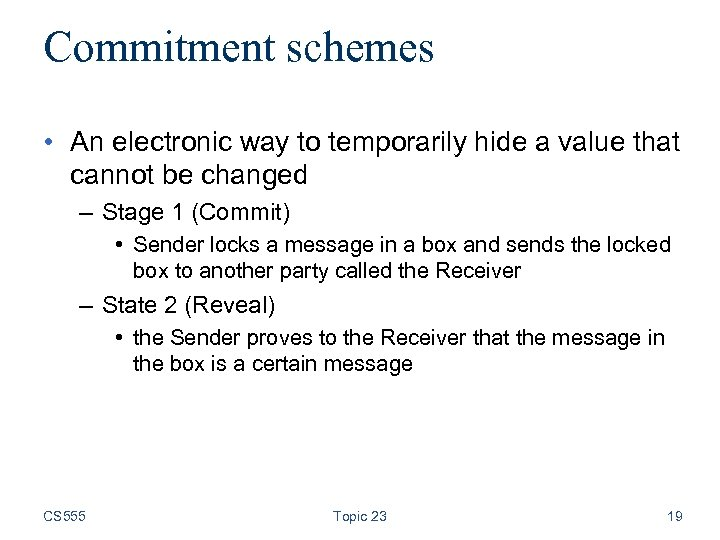Commitment schemes • An electronic way to temporarily hide a value that cannot be changed – Stage 1 (Commit) • Sender locks a message in a box and sends the locked box to another party called the Receiver – State 2 (Reveal) • the Sender proves to the Receiver that the message in the box is a certain message CS 555 Topic 23 19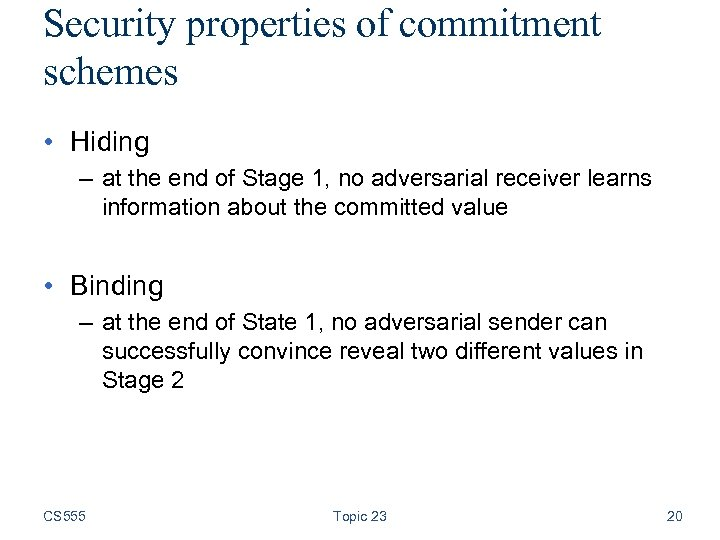Security properties of commitment schemes • Hiding – at the end of Stage 1, no adversarial receiver learns information about the committed value • Binding – at the end of State 1, no adversarial sender can successfully convince reveal two different values in Stage 2 CS 555 Topic 23 20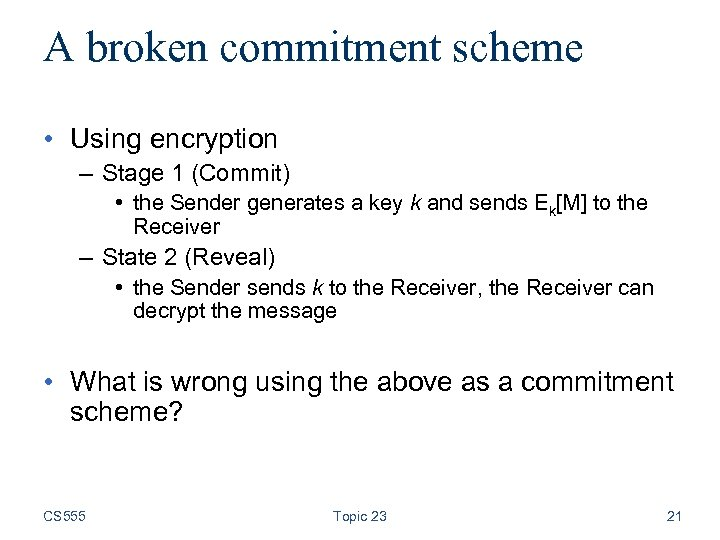A broken commitment scheme • Using encryption – Stage 1 (Commit) • the Sender generates a key k and sends Ek[M] to the Receiver – State 2 (Reveal) • the Sender sends k to the Receiver, the Receiver can decrypt the message • What is wrong using the above as a commitment scheme? CS 555 Topic 23 21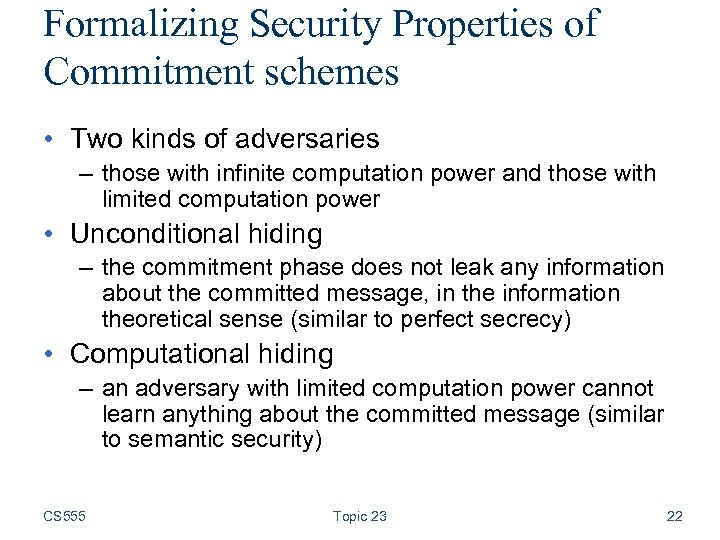Formalizing Security Properties of Commitment schemes • Two kinds of adversaries – those with infinite computation power and those with limited computation power • Unconditional hiding – the commitment phase does not leak any information about the committed message, in the information theoretical sense (similar to perfect secrecy) • Computational hiding – an adversary with limited computation power cannot learn anything about the committed message (similar to semantic security) CS 555 Topic 23 22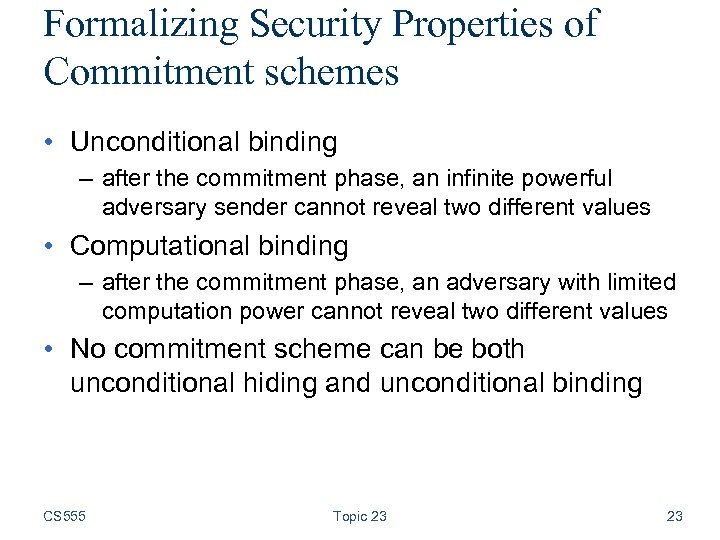Formalizing Security Properties of Commitment schemes • Unconditional binding – after the commitment phase, an infinite powerful adversary sender cannot reveal two different values • Computational binding – after the commitment phase, an adversary with limited computation power cannot reveal two different values • No commitment scheme can be both unconditional hiding and unconditional binding CS 555 Topic 23 23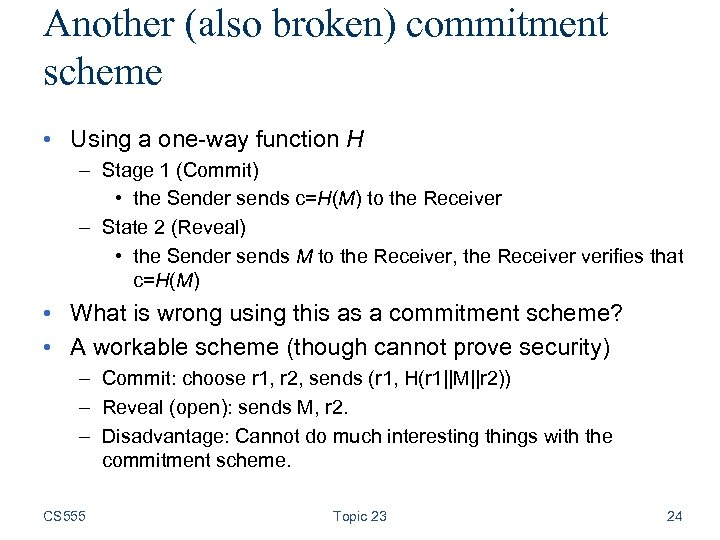Another (also broken) commitment scheme • Using a one-way function H – Stage 1 (Commit) • the Sender sends c=H(M) to the Receiver – State 2 (Reveal) • the Sender sends M to the Receiver, the Receiver verifies that c=H(M) • What is wrong using this as a commitment scheme? • A workable scheme (though cannot prove security) – Commit: choose r 1, r 2, sends (r 1, H(r 1||M||r 2)) – Reveal (open): sends M, r 2. – Disadvantage: Cannot do much interesting things with the commitment scheme. CS 555 Topic 23 24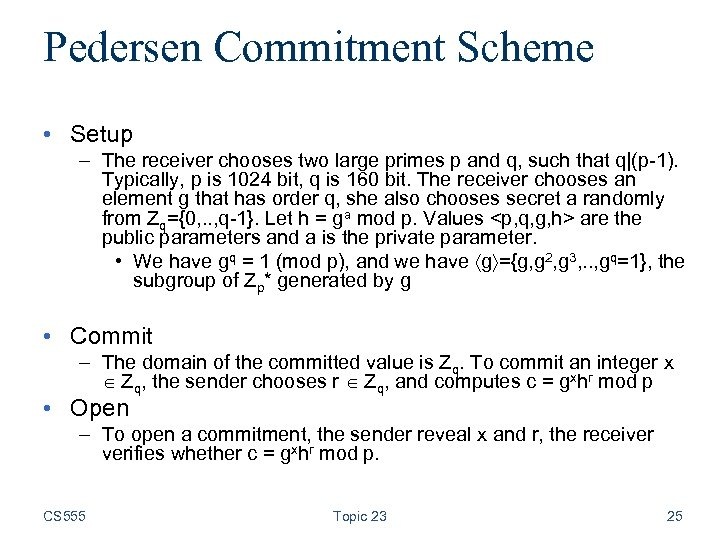Pedersen Commitment Scheme • Setup – The receiver chooses two large primes p and q, such that q|(p-1). Typically, p is 1024 bit, q is 160 bit. The receiver chooses an element g that has order q, she also chooses secret a randomly from Zq={0, . . , q-1}. Let h = ga mod p. Values are the public parameters and a is the private parameter. • We have gq = 1 (mod p), and we have g ={g, g 2, g 3, . . , gq=1}, the subgroup of Zp* generated by g • Commit – The domain of the committed value is Zq. To commit an integer x Zq, the sender chooses r Zq, and computes c = gxhr mod p • Open – To open a commitment, the sender reveal x and r, the receiver verifies whether c = gxhr mod p. CS 555 Topic 23 25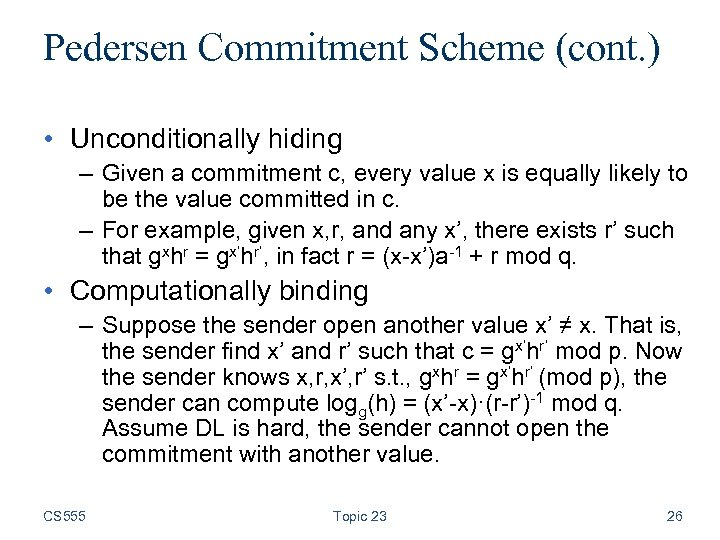Pedersen Commitment Scheme (cont. ) • Unconditionally hiding – Given a commitment c, every value x is equally likely to be the value committed in c. – For example, given x, r, and any x’, there exists r’ such that gxhr = gx’hr’, in fact r = (x-x’)a-1 + r mod q. • Computationally binding – Suppose the sender open another value x’ ≠ x. That is, the sender find x’ and r’ such that c = gx’hr’ mod p. Now the sender knows x, r, x’, r’ s. t. , gxhr = gx’hr’ (mod p), the sender can compute logg(h) = (x’-x)·(r-r’)-1 mod q. Assume DL is hard, the sender cannot open the commitment with another value. CS 555 Topic 23 26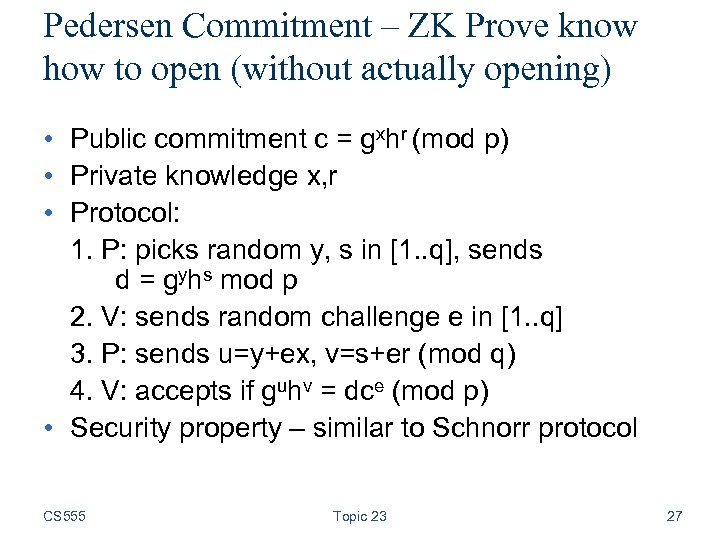Pedersen Commitment – ZK Prove know how to open (without actually opening) • Public commitment c = gxhr (mod p) • Private knowledge x, r • Protocol: 1. P: picks random y, s in [1. . q], sends d = gyhs mod p 2. V: sends random challenge e in [1. . q] 3. P: sends u=y+ex, v=s+er (mod q) 4. V: accepts if guhv = dce (mod p) • Security property – similar to Schnorr protocol CS 555 Topic 23 27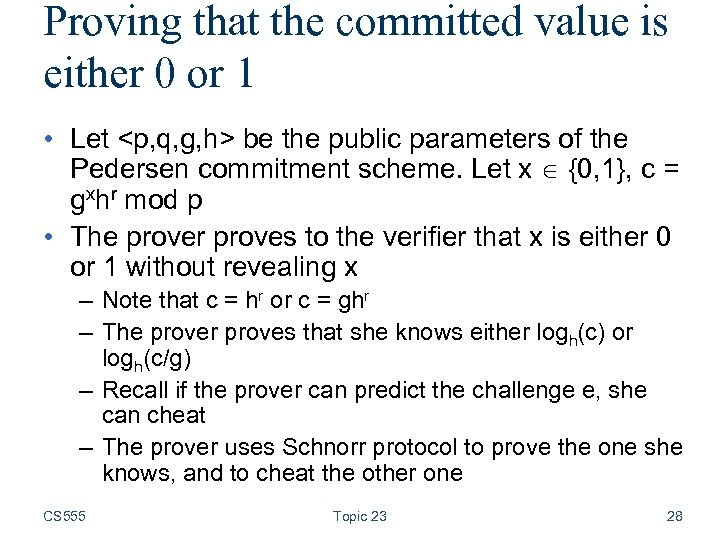Proving that the committed value is either 0 or 1 • Let be the public parameters of the Pedersen commitment scheme. Let x {0, 1}, c = gxhr mod p • The prover proves to the verifier that x is either 0 or 1 without revealing x – Note that c = hr or c = ghr – The prover proves that she knows either logh(c) or logh(c/g) – Recall if the prover can predict the challenge e, she can cheat – The prover uses Schnorr protocol to prove the one she knows, and to cheat the other one CS 555 Topic 23 28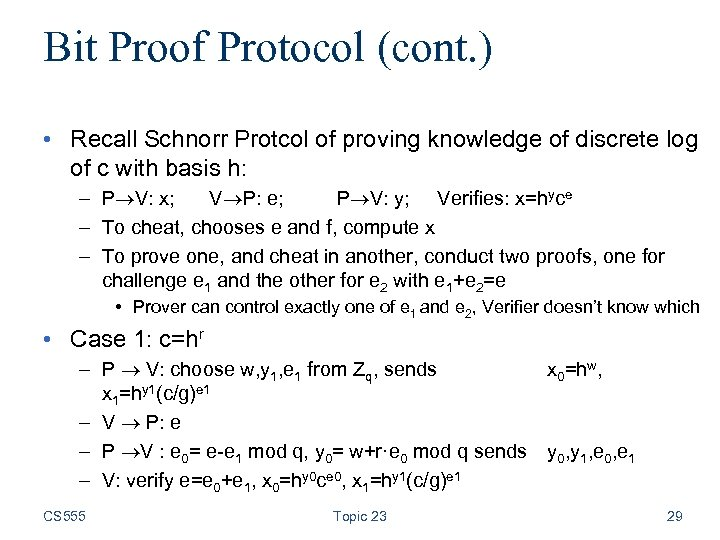Bit Proof Protocol (cont. ) • Recall Schnorr Protcol of proving knowledge of discrete log of c with basis h: – P V: x; V P: e; P V: y; Verifies: x=hyce – To cheat, chooses e and f, compute x – To prove one, and cheat in another, conduct two proofs, one for challenge e 1 and the other for e 2 with e 1+e 2=e • Prover can control exactly one of e 1 and e 2, Verifier doesn’t know which • Case 1: c=hr – P V: choose w, y 1, e 1 from Zq, sends x 0=hw, x 1=hy 1(c/g)e 1 – V P: e – P V : e 0= e-e 1 mod q, y 0= w+r·e 0 mod q sends y 0, y 1, e 0, e 1 – V: verify e=e 0+e 1, x 0=hy 0 ce 0, x 1=hy 1(c/g)e 1 CS 555 Topic 23 29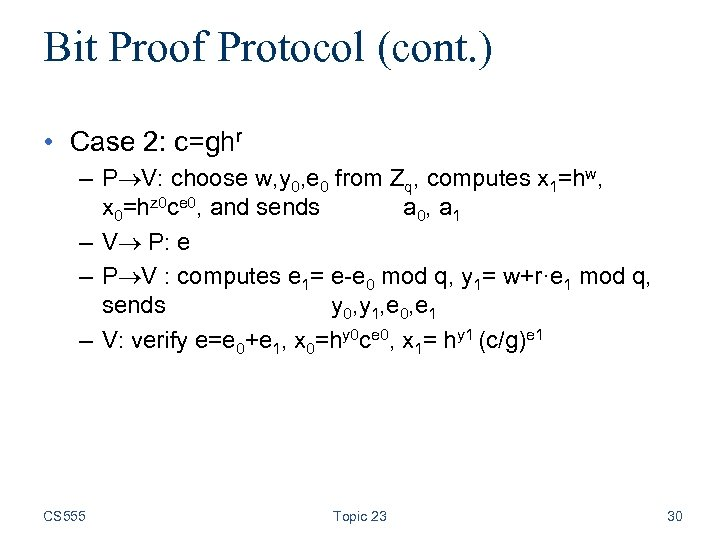Bit Proof Protocol (cont. ) • Case 2: c=ghr – P V: choose w, y 0, e 0 from Zq, computes x 1=hw, x 0=hz 0 ce 0, and sends a 0, a 1 – V P: e – P V : computes e 1= e-e 0 mod q, y 1= w+r·e 1 mod q, sends y 0, y 1, e 0, e 1 – V: verify e=e 0+e 1, x 0=hy 0 ce 0, x 1= hy 1 (c/g)e 1 CS 555 Topic 23 30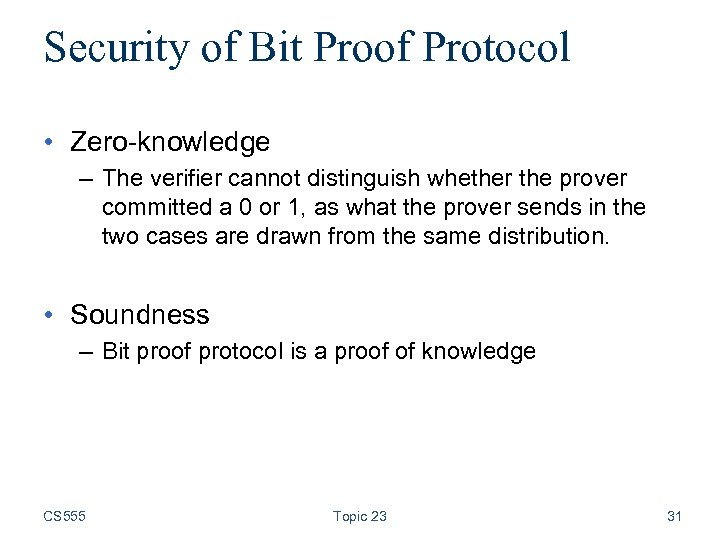Security of Bit Proof Protocol • Zero-knowledge – The verifier cannot distinguish whether the prover committed a 0 or 1, as what the prover sends in the two cases are drawn from the same distribution. • Soundness – Bit proof protocol is a proof of knowledge CS 555 Topic 23 31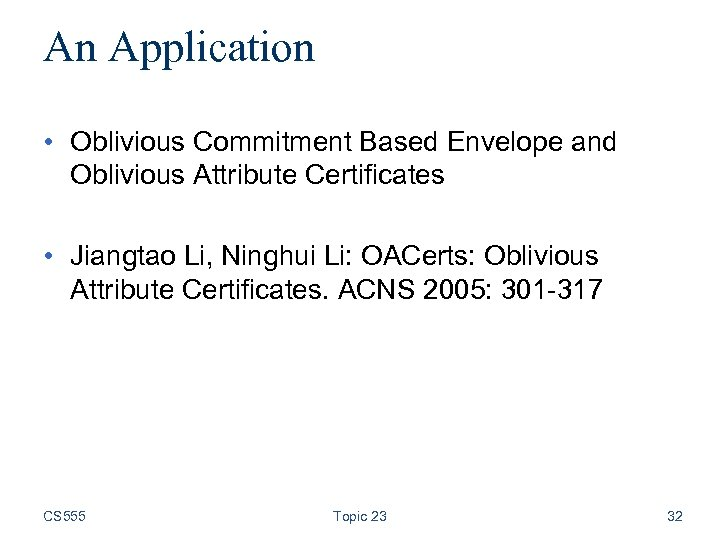An Application • Oblivious Commitment Based Envelope and Oblivious Attribute Certificates • Jiangtao Li, Ninghui Li: OACerts: Oblivious Attribute Certificates. ACNS 2005: 301 -317 CS 555 Topic 23 32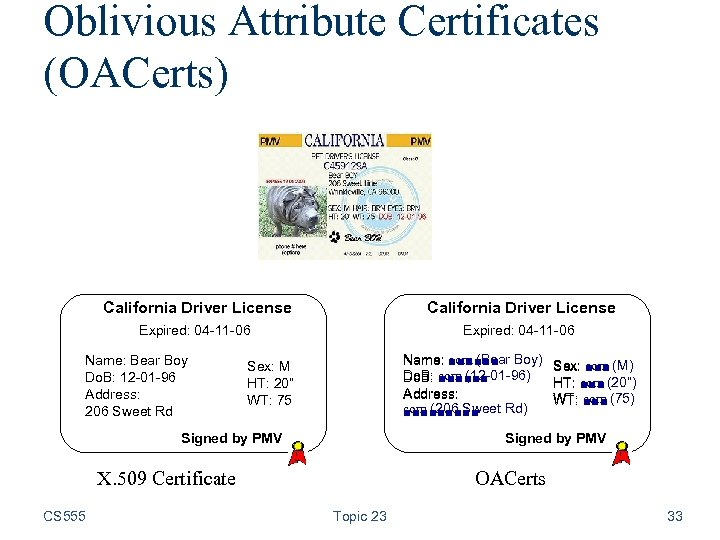Oblivious Attribute Certificates (OACerts) California Driver License Expired: 04 -11 -06 Name: Bear Boy Do. B: 12 -01 -96 Address: 206 Sweet Rd Name: com (Bear Boy) ■■■■■■ Do. B: com (12 -01 -96) ■■■■■■ Address: com (206 Sweet Rd) ■■■■■ Sex: M HT: 20” WT: 75 Signed by PMV X. 509 Certificate CS 555 Sex: com (M) ■■■ HT: com (20”) ■■■ WT: com (75) ■■■ OACerts Topic 23 33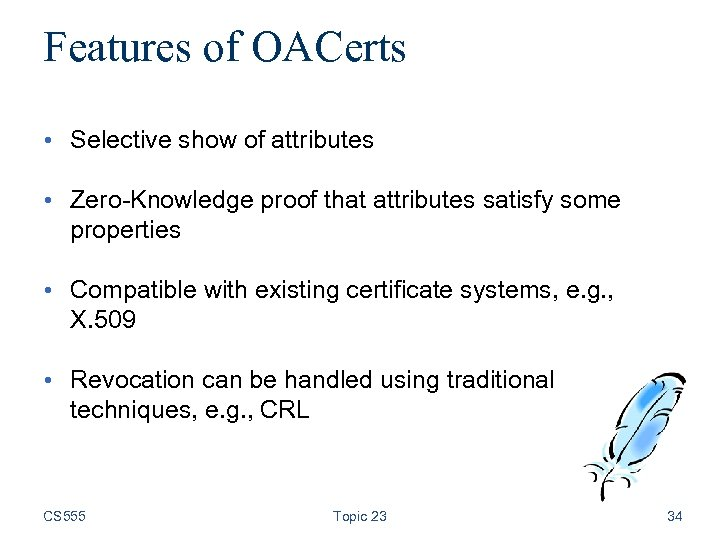Features of OACerts • Selective show of attributes • Zero-Knowledge proof that attributes satisfy some properties • Compatible with existing certificate systems, e. g. , X. 509 • Revocation can be handled using traditional techniques, e. g. , CRL CS 555 Topic 23 34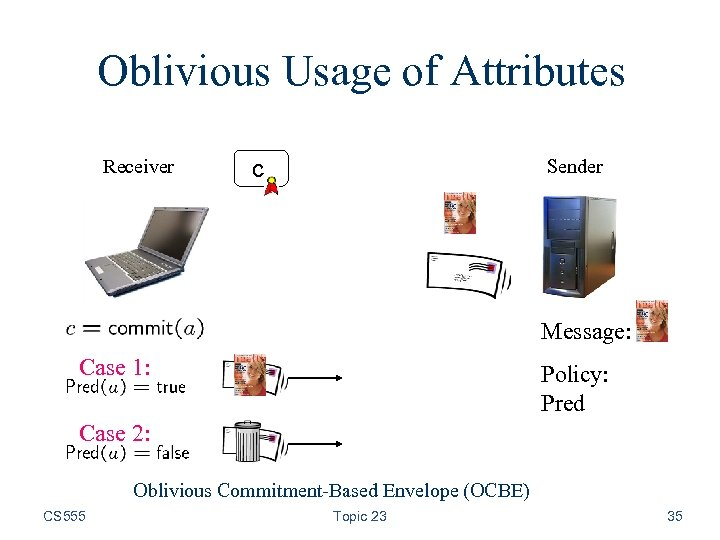Oblivious Usage of Attributes Receiver Sender c Message: Case 1: Policy: Pred Case 2: Oblivious Commitment-Based Envelope (OCBE) CS 555 Topic 23 35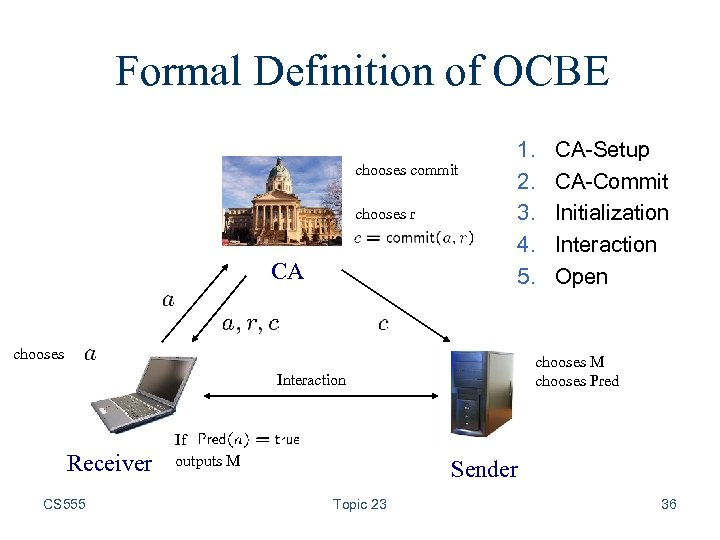Formal Definition of OCBE chooses commit chooses r CA 1. 2. 3. 4. 5. chooses M chooses Pred Interaction Receiver CS 555 If outputs M CA-Setup CA-Commit Initialization Interaction Open Sender Topic 23 36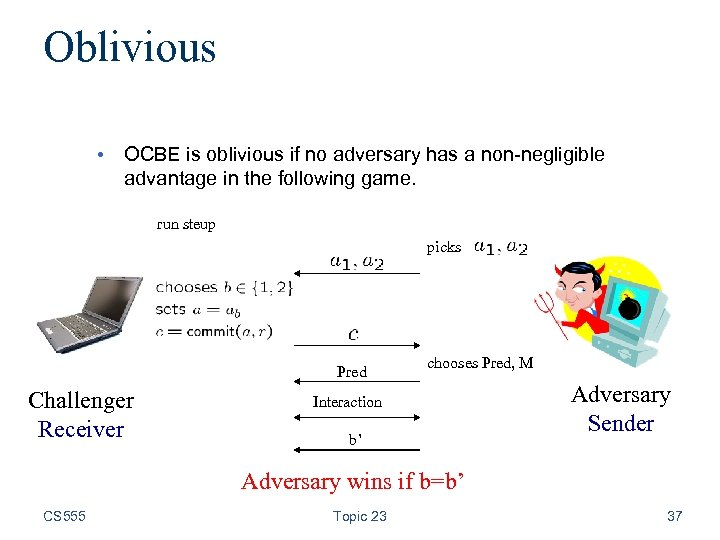Oblivious • OCBE is oblivious if no adversary has a non-negligible advantage in the following game. run steup picks Pred Challenger Receiver chooses Pred, M Interaction b’ Adversary Sender Adversary wins if b=b’ CS 555 Topic 23 37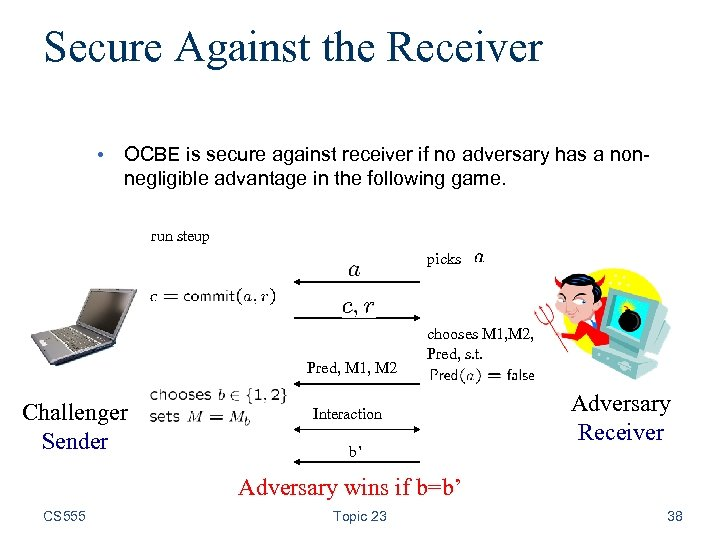Secure Against the Receiver • OCBE is secure against receiver if no adversary has a nonnegligible advantage in the following game. run steup picks Pred, M 1, M 2 Challenger Sender chooses M 1, M 2, Pred, s. t. Interaction b’ Adversary Receiver Adversary wins if b=b’ CS 555 Topic 23 38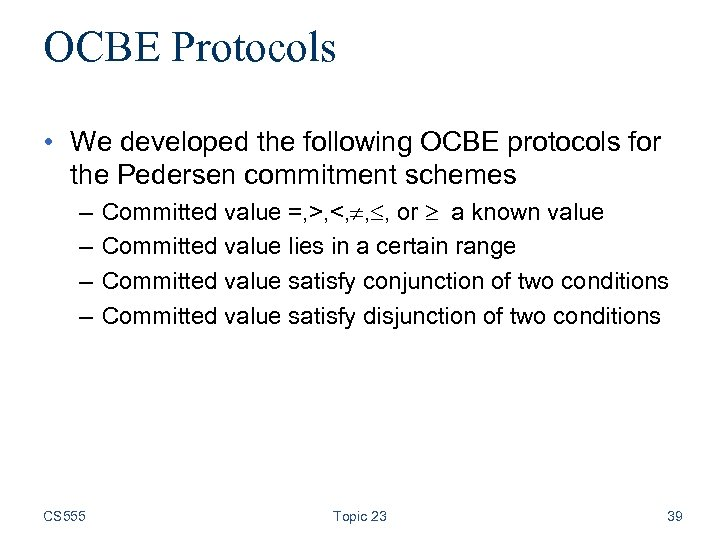OCBE Protocols • We developed the following OCBE protocols for the Pedersen commitment schemes – – CS 555 Committed value =, >, <, , , or a known value Committed value lies in a certain range Committed value satisfy conjunction of two conditions Committed value satisfy disjunction of two conditions Topic 23 39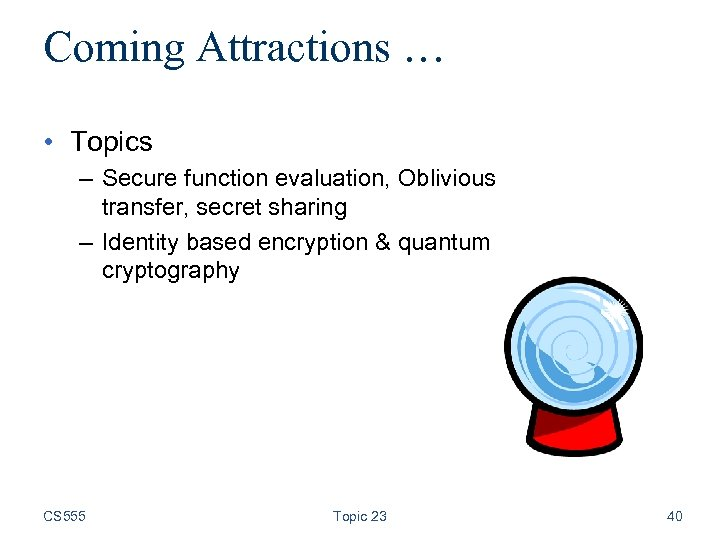Coming Attractions … • Topics – Secure function evaluation, Oblivious transfer, secret sharing – Identity based encryption & quantum cryptography CS 555 Topic 23 40